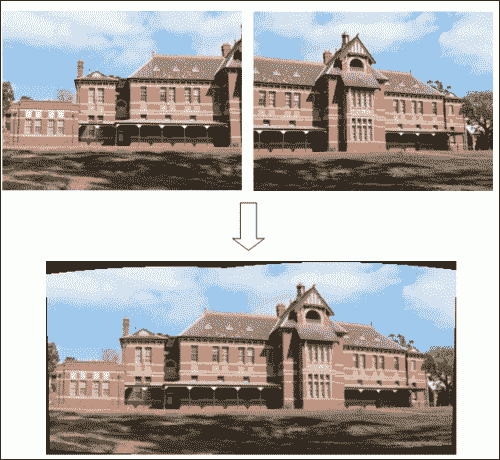• 视频稳定
• 视频超分辨率过程
• 图像拼接

# 视频稳定

• 机械稳定系统：这些系统在摄像机镜头上使用机械系统，因此在移动摄像机时，加速度计和陀螺仪会检测到运动，并且系统会在运动时产生运动。 镜片。 这些系统将不在此处考虑。
• 数字稳定系统：这些通常是视频中使用的，它们直接作用于从摄像机获得的图像。 在这些系统中，稳定图像的表面略小于源图像的表面。 移动相机时，拍摄的图像也会移动以补偿该移动。 尽管这些技术通过减小运动传感器的可用面积有效地消除了运动，但是却牺牲了分辨率和图像清晰度。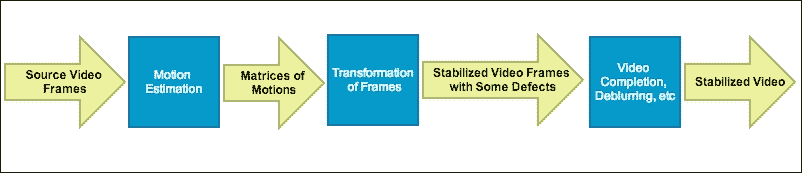### 注意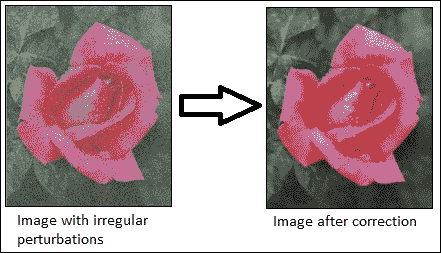#include <string>
#include <iostream>
#include <opencv2/opencv.hpp>
#include <opencv2/videostab.hpp>

using namespace std;
using namespace cv;
using namespace cv::videostab;

void processing(Ptr<IFrameSource> stabilizedFrames, string outputPath);

int main(int argc, const char **argv)
{
Ptr<IFrameSource> stabilizedFrames;
try
{

// 1-Prepare the input video and check it
string inputPath;
string outputPath;
if (argc > 1)
inputPath = argv;
else
inputPath = ".\\cube4.avi";

if (argc > 2)
outputPath = argv;
else
outputPath = ".\\cube4_stabilized.avi";

Ptr<VideoFileSource> source = makePtr<VideoFileSource>(inputPath);
cout << "frame count (rough): " << source->count() << endl;

// 2-Prepare the motion estimator
// first, prepare the motion the estimation builder, RANSAC L2
double min_inlier_ratio = 0.1;
Ptr<MotionEstimatorRansacL2> est = makePtr<MotionEstimatorRansacL2>(MM_AFFINE);
RansacParams ransac = est->ransacParams();
ransac.size = 3;
ransac.thresh = 5;
ransac.eps = 0.5;
est->setRansacParams(ransac);
est->setMinInlierRatio(min_inlier_ratio);

// second, create a feature detector
int nkps = 1000;
Ptr<GoodFeaturesToTrackDetector> feature_detector = makePtr<GoodFeaturesToTrackDetector>(nkps);

// third, create the motion estimator
Ptr<KeypointBasedMotionEstimator> motionEstBuilder = makePtr<KeypointBasedMotionEstimator>(est);
motionEstBuilder->setDetector(feature_detector);
Ptr<IOutlierRejector> outlierRejector = makePtr<NullOutlierRejector>();
motionEstBuilder->setOutlierRejector(outlierRejector);

// 3-Prepare the stabilizer
StabilizerBase *stabilizer = 0;

// first, prepare the one or two pass stabilizer
bool isTwoPass = 1;
if (isTwoPass)
{
// with a two pass stabilizer
bool est_trim = true;

TwoPassStabilizer *twoPassStabilizer = new TwoPassStabilizer();
twoPassStabilizer->setEstimateTrimRatio(est_trim);

stabilizer = twoPassStabilizer;
}
else
{
// with an one pass stabilizer
OnePassStabilizer *onePassStabilizer = new OnePassStabilizer();

stabilizer = onePassStabilizer;
}

// second, set up the parameters
double trim_ratio = 0.1;
bool incl_constr = false;
stabilizer->setFrameSource(source);
stabilizer->setMotionEstimator(motionEstBuilder);
stabilizer->setTrimRatio(trim_ratio);
stabilizer->setCorrectionForInclusion(incl_constr);
stabilizer->setBorderMode(BORDER_REPLICATE);

// cast stabilizer to simple frame source interface to read stabilized frames
stabilizedFrames.reset(dynamic_cast<IFrameSource*>(stabilizer));

// 4-Processing the stabilized frames. The results are showed and saved.
processing(stabilizedFrames, outputPath);
}
catch (const exception &e)
{
cout << "error: " << e.what() << endl;
stabilizedFrames.release();
return -1;
}
stabilizedFrames.release();
return 0;
}

void processing(Ptr<IFrameSource> stabilizedFrames, string outputPath)
{
VideoWriter writer;
Mat stabilizedFrame;
int nframes = 0;
double outputFps = 25;

// for each stabilized frame
while (!(stabilizedFrame = stabilizedFrames->nextFrame()).empty())
{
nframes++;

// init writer (once) and save stabilized frame
if (!outputPath.empty())
{
if (!writer.isOpened())                writer.open(outputPath,VideoWriter::fourcc('X','V','I','D'),
outputFps, stabilizedFrame.size());
writer << stabilizedFrame;
}

imshow("stabilizedFrame", stabilizedFrame);
char key = static_cast<char>(waitKey(3));
if (key == 27) { cout << endl; break;}

}
cout << "processed frames: " << nframes << endl;
cout << "finished " << endl;
}


• MM_TRANSLATION = 0
• MM_TRANSLATION_AND_SCALE = 1
• MM_ROTATIO = 2
• MM_RIGID = 3
• MM_SIMILARITY = 4
• MM_AFFINE = 5
• MM_HOMOGRAPHY = 6
• MM_UNKNOWN = 7

RANSAC 参数
size
eps
thresh
prob

<bin_dir>\videoStabilizer.exe .\cube4.avi .\cube4_stabilized.avi



### 注意

cube4.avi视频可在 OpenCV 示例文件夹中找到。 它还具有大量的相机移动，这对于此示例来说是完美的。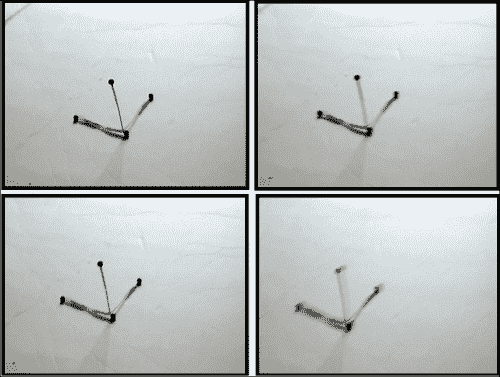cube4.avi视频的四个连续帧是摄像机的移动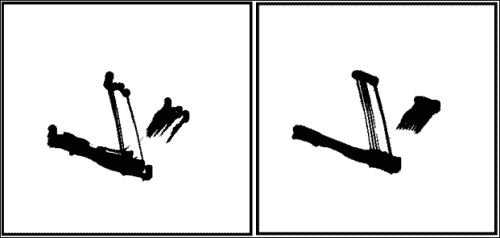cube4.avicube4_stabilizated视频的 10 个叠加帧

# 超分辨率

• 采样：这是连续系统从理想离散系统场景开始的变换，没有混叠
• 几何变换：此是指根据摄像机的位置和镜头系统应用一组变换（例如平移或旋转）来理想地推断到达每个传感器的场景细节
• 模糊：这是由于镜头系统或积分期间场景中的现有运动造成的
• 二次采样：传感器仅对可使用的像素数（照片）进行积分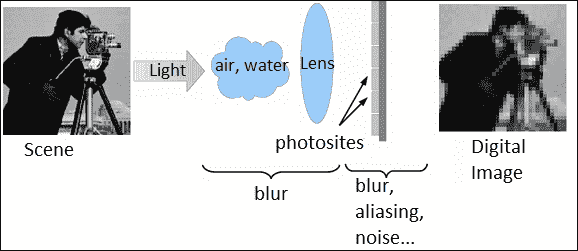### 注意

#include <iostream>
#include <iomanip>
#include <string>

#include <opencv2/core.hpp>
#include <opencv2/core/utility.hpp>
#include <opencv2/highgui.hpp>
#include <opencv2/imgproc.hpp>
#include <opencv2/superres.hpp>
#include <opencv2/superres/optical_flow.hpp>
#include <opencv2/opencv_modules.hpp>

using namespace std;
using namespace cv;
using namespace cv::superres;

static Ptr<DenseOpticalFlowExt> createOptFlow(string name);

int main(int argc, char *argv[])
{
// 1-Initialize the initial parameters

// Input and output video
string inputVideoName;
string outputVideoName;
if (argc > 1)
inputVideoName = argv;
else
inputVideoName = ".\\tree.avi";

if (argc > 2)
outputVideoName = argv;
else
outputVideoName = ".\\tree_superresolution.avi";

const int scale = 4;// Scale factor

const int iterations = 180;// Iterations count

string optFlow = "farneback";// Optical flow algorithm
// optFlow = "farneback";
// optFlow = "tvl1";
// optFlow = "brox";
// optFlow = "pyrlk";

double outputFps = 25.0;// Playback speed output

// 2- Create an optical flow method
Ptr<DenseOpticalFlowExt> optical_flow = createOptFlow(optFlow);

if (optical_flow.empty()) return -1;

// 3- Create the superresolution method and set its parameters
Ptr<SuperResolution> superRes;
superRes = createSuperResolution_BTVL1();

superRes->set("opticalFlow", optical_flow);
superRes->set("scale", scale);
superRes->set("iterations", iterations);

Ptr<FrameSource> frameSource;
frameSource = createFrameSource_Video(inputVideoName);

superRes->setInput(frameSource);

// Not use the first frame
Mat frame;
frameSource->nextFrame(frame);

// 4- Processing the input video with the superresolution
// Show the initial options
cout << "Input                  : " << inputVideoName << " " << frame.size() << endl;
cout << "Output                 : " << outputVideoName << endl;
cout << "Playback speed output  : " << outputFps << endl;
cout << "Scale factor           : " << scale << endl;
cout << "Iterations             : " << iterations << endl;
cout << "Optical Flow           : " << optFlow << endl;
cout << endl;

VideoWriter writer;
double start_time,finish_time;

for (int i = 0;; ++i)
{
cout << '[' << setw(3) << i << "] : ";
Mat result;

// Calculate the processing time
start_time = getTickCount();
superRes->nextFrame(result);
finish_time = getTickCount();
cout << (finish_time - start_time)/getTickFrequency() << " secs, Size: " << result.size() << endl;

if (result.empty()) break;

// Show the result
imshow("Super Resolution", result);

if (waitKey(1000) > 0) break;

// Save the result on output file
if (!outputVideoName.empty())
{
if (!writer.isOpened())
writer.open(outputVideoName, VideoWriter::fourcc('X', 'V', 'I', 'D'), outputFps, result.size());
writer << result;
}
}
writer.release();
return 0;
}

static Ptr<DenseOpticalFlowExt> createOptFlow(string name)
{
if (name == "farneback")
return createOptFlow_Farneback();

else if (name == "tvl1")
return createOptFlow_DualTVL1();

else if (name == "brox")
return createOptFlow_Brox_CUDA();

else if (name == "pyrlk")
return createOptFlow_PyrLK_CUDA();

else
cerr << "Incorrect Optical Flow algorithm - " << name << endl;

return Ptr<DenseOpticalFlowExt>();
}


Farneback 二次
TVL1 线性
Brox 线性
Pyrlk 线性

### 注意

• scale：这是比例因子
• iterations：这是迭代计数
• tau：这是最速下降法的渐近值
• lambda：这是权重参数，用于平衡数据项和平滑度项
• alpha：这是双边电视中空间分布的参数
• btvKernelSize：这是双边电视过滤器的核大小
• blurKernelSize：这是高斯模糊核大小
• blurSigma：这是高斯模糊西格玛
• temporalAreaRadius：这是时间搜索区域的半径
• opticalFlow：这是一种密集光流算法

superRes->set("parameter", value);


superRes->set("opticalFlow", optical_flow);
superRes->set("scale", scale);
superRes->set("iterations", iterations);tree.avitree_superresolution.avi视频的第一帧的一部分，不包括超分辨率处理

# 拼接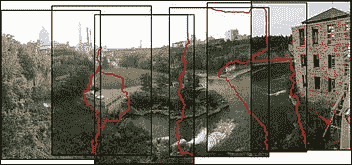### 注意

• 配准（图像）表示一组图像中的匹配特征以搜索使重叠像素之间差异的绝对值之和最小的位移。 直接比对方法可用于获得更好的结果。 用户还可以添加全景图的粗略模型以帮助特征匹配阶段，在这种情况下，结果通常更准确且计算速度更快。
• 校准（图像）将重点放在最小化理想模型和相机镜头系统之间的差异：不同的相机位置和光学缺陷，例如失真，曝光，色差等。
• 合成（图像）使用上一步校准的结果，并将图像重新映射到输出投影。 图像之间的颜色也会进行调整，以补偿曝光差异。 将图像融合在一起，并进行接缝线调整以最小化图像之间接缝的可见性。

• 直线投影：在这里，在与全景球在一个点相交的二维平面上查看拼接图像。 无论图像上的方向如何，现实中笔直的线都显示类似。 当视野开阔（大约 120 度）时，图像在边框附近会变形。
• 圆柱投影：此处，拼接图像显示 360 度水平视场和有限的垂直视场。 该投影旨在被视为好像图像被包裹在圆柱体中并从内部观看。 在 2D 平面上查看时，水平线看起来是弯曲的，而垂直线则保持笔直。
• 球形投影：在这里，拼接图像显示了 360 度水平视野和 180 度垂直视野，即整个球体。 具有这种投影的全景图像旨在像被包裹在一个球体中并从内部观看一样被观看。 在 2D 平面上查看时，水平线看起来像圆柱投影一样弯曲，而垂直线则保持垂直。
• 立体投影或鱼眼投影：通过将虚拟摄像机指向下方并将视场设置为足够大以显示整个地面及其上方的某些区域，可以将其用于形成一个小行星全景。 将虚拟摄像机指向上方会产生隧道效果。
• 帕尼尼投影：这具有专业投影，可能比常规制图投影在美学上更具优势。 此投影将同一图像中的不同投影组合在一起，以微调输出全景图像的最终外观。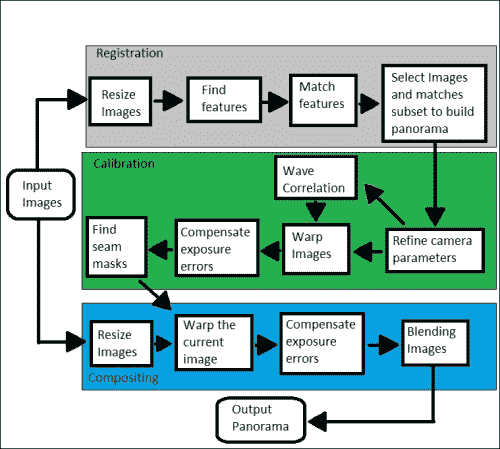#include <iostream>
#include <string>
#include <opencv2/opencv_modules.hpp>
#include <opencv2/core/utility.hpp>
#include <opencv2/imgcodecs.hpp>
#include <opencv2/highgui.hpp>
#include <opencv2/features2d.hpp>
#include <opencv2/stitching/detail/blenders.hpp>
#include <opencv2/stitching/detail/camera.hpp>
#include <opencv2/stitching/detail/exposure_compensate.hpp>
#include <opencv2/stitching/detail/matchers.hpp>
#include <opencv2/stitching/detail/motion_estimators.hpp>
#include <opencv2/stitching/detail/seam_finders.hpp>
#include <opencv2/stitching/detail/util.hpp>
#include <opencv2/stitching/detail/warpers.hpp>
#include <opencv2/stitching/warpers.hpp>

using namespace std;
using namespace cv;
using namespace cv::detail;

int main(int argc, char* argv[])
{
// Default parameters
vector<String> img_names;
double scale = 1;
string features_type = "orb";//"surf" or "orb" features type
float match_conf = 0.3f;
float conf_thresh = 1.f;
bool do_wave_correct = true;
WaveCorrectKind wave_correct_type = WAVE_CORRECT_HORIZ;
string warp_type = "spherical";
int expos_comp_type = ExposureCompensator::GAIN_BLOCKS;
string seam_find_type = "gc_color";
float blend_strength = 5;
int blend_type = Blender::MULTI_BAND;
string result_name = "panorama_result.jpg";

double start_time = getTickCount();

// 1-Input images
if(argc > 1)
{
for(int i=1; i < argc; i++)
img_names.push_back(argv[i]);
}
else
{
img_names.push_back("./panorama_image1.jpg");
img_names.push_back("./panorama_image2.jpg");
}
// Check if have enough images
int num_images = static_cast<int>(img_names.size());
if (num_images < 2) {cout << "Need more images" << endl; return -1; }

// 2- Resize images and find features steps
cout << "Finding features..." << endl;
double t = getTickCount();

Ptr<FeaturesFinder> finder;
if (features_type == "surf")
finder = makePtr<SurfFeaturesFinder>();

else if (features_type == "orb")
finder = makePtr<OrbFeaturesFinder>();

else {cout << "Unknown 2D features type: '" << features_type << endl; return -1; }

Mat full_img, img;
vector<ImageFeatures> features(num_images);
vector<Mat> images(num_images);
vector<Size> full_img_sizes(num_images);

for (int i = 0; i < num_images; ++i)
{
full_img_sizes[i] = full_img.size();

if (full_img.empty()) {cout << "Can't open image " << img_names[i] << endl; return -1; }

resize(full_img, img, Size(), scale, scale);
images[i] = img.clone();

(*finder)(img, features[i]);
features[i].img_idx = i;
cout << "Features in image #" << i+1 << " are : " << features[i].keypoints.size() << endl;
}
finder->collectGarbage();
full_img.release();
img.release();
cout << "Finding features, time: " << ((getTickCount() - t) / getTickFrequency()) << " sec" << endl;

// 3- Match features
cout << "Pairwise matching" << endl;
t = getTickCount();
vector<MatchesInfo> pairwise_matches;
BestOf2NearestMatcher matcher(false, match_conf);
matcher(features, pairwise_matches);
matcher.collectGarbage();
cout << "Pairwise matching, time: " << ((getTickCount() - t) / getTickFrequency()) << " sec" << endl;

// 4- Select images and matches subset to build panorama
vector<int> indices = leaveBiggestComponent(features, pairwise_matches, conf_thresh);
vector<Mat> img_subset;
vector<String> img_names_subset;
vector<Size> full_img_sizes_subset;
for (size_t i = 0; i < indices.size(); ++i)
{
img_names_subset.push_back(img_names[indices[i]]);
img_subset.push_back(images[indices[i]]);
full_img_sizes_subset.push_back(full_img_sizes[indices[i]]);
}
images = img_subset;
img_names = img_names_subset;
full_img_sizes = full_img_sizes_subset;

// Estimate camera parameters rough
HomographyBasedEstimator estimator;
vector<CameraParams> cameras;
if (!estimator(features, pairwise_matches, cameras)){cout << "Homography estimation failed." << endl; return -1; }

for (size_t i = 0; i < cameras.size(); ++i)
{
Mat R;
cameras[i].R.convertTo(R, CV_32F);
cameras[i].R = R;
cout << "Initial intrinsic #" << indices[i]+1 << ":\n" << cameras[i].K() << endl;
}

// 5- Refine camera parameters globally
// "reproj" method
else // "ray" method

if (!(*adjuster)(features, pairwise_matches, cameras)) {cout << "Camera parameters adjusting failed." << endl; return -1; }

// Find median focal length
vector<double> focals;
for (size_t i = 0; i < cameras.size(); ++i)
{
cout << "Camera #" << indices[i]+1 << ":\n" << cameras[i].K() << endl;
focals.push_back(cameras[i].focal);
}
sort(focals.begin(), focals.end());
float warped_image_scale;
if (focals.size() % 2 == 1)
warped_image_scale = static_cast<float>(focals[focals.size() / 2]);
else
warped_image_scale = static_cast<float>(focals[focals.size() / 2 - 1] + focals[focals.size() / 2]) * 0.5f;

// 6- Wave correlation (optional)
if (do_wave_correct)
{
vector<Mat> rmats;
for (size_t i = 0; i < cameras.size(); ++i)
rmats.push_back(cameras[i].R.clone());

waveCorrect(rmats, wave_correct_type);
for (size_t i = 0; i < cameras.size(); ++i)
cameras[i].R = rmats[i];
}

// 7- Warp images
cout << "Warping images (auxiliary)... " << endl;
t = getTickCount();
vector<Point> corners(num_images);
vector<UMat> images_warped(num_images);
vector<Size> sizes(num_images);

for (int i = 0; i < num_images; ++i)
{
}

// Map projections
Ptr<WarperCreator> warper_creator;
if (warp_type == "rectilinear")
warper_creator = makePtr<cv::CompressedRectilinearWarper>(2.0f, 1.0f);

else if (warp_type == "cylindrical")
warper_creator = makePtr<cv::CylindricalWarper>();

else if (warp_type == "spherical")
warper_creator = makePtr<cv::SphericalWarper>();

else if (warp_type == "stereographic")
warper_creator = makePtr<cv::StereographicWarper>();

else if (warp_type == "panini")
warper_creator = makePtr<cv::PaniniWarper>(2.0f, 1.0f);

if (!warper_creator){ cout << "Can't create the following warper '" << warp_type << endl; return 1; }

Ptr<RotationWarper> warper = warper_creator->create(static_cast<float>(warped_image_scale * scale));

for (int i = 0; i < num_images; ++i)
{
Mat_<float> K;
cameras[i].K().convertTo(K, CV_32F);
float swa = (float)scale;
K(0,0) *= swa; K(0,2) *= swa;
K(1,1) *= swa; K(1,2) *= swa;

corners[i] = warper->warp(images[i], K, cameras[i].R, INTER_LINEAR, BORDER_REFLECT, images_warped[i]);
sizes[i] = images_warped[i].size();

}

vector<UMat> images_warped_f(num_images);
for (int i = 0; i < num_images; ++i)
images_warped[i].convertTo(images_warped_f[i], CV_32F);

cout << "Warping images, time: " << ((getTickCount() - t) / getTickFrequency()) << " sec" << endl;

// 8- Compensate exposure errors
Ptr<ExposureCompensator> compensator = ExposureCompensator::createDefault(expos_comp_type);

Ptr<SeamFinder> seam_finder;
if (seam_find_type == "no")
seam_finder = makePtr<NoSeamFinder>();

else if (seam_find_type == "voronoi")
seam_finder = makePtr<VoronoiSeamFinder>();

else if (seam_find_type == "gc_color")
seam_finder = makePtr<GraphCutSeamFinder>(GraphCutSeamFinderBase::COST_COLOR);

else if (seam_find_type == "dp_color")
seam_finder = makePtr<DpSeamFinder>(DpSeamFinder::COLOR);

if (!seam_finder){cout << "Can't create the following seam finder '" << seam_find_type << endl; return 1; }

// Release unused memory
images.clear();
images_warped.clear();
images_warped_f.clear();

// 10- Create a blender
Ptr<Blender> blender = Blender::createDefault(blend_type, false);
Size dst_sz = resultRoi(corners, sizes).size();
float blend_width = sqrt(static_cast<float>(dst_sz.area())) * blend_strength / 100.f;
if (blend_width < 1.f)
blender = Blender::createDefault(Blender::NO, false);

else if (blend_type == Blender::MULTI_BAND)
{
MultiBandBlender* mb = dynamic_cast<MultiBandBlender*>(blender.get());
mb->setNumBands(static_cast<int>(ceil(log(blend_width)/log(2.)) - 1.));
cout << "Multi-band blender, number of bands: " << mb->numBands() << endl;
}
else if (blend_type == Blender::FEATHER)
{
FeatherBlender* fb = dynamic_cast<FeatherBlender*>(blender.get());
fb->setSharpness(1.f/blend_width);
cout << "Feather blender, sharpness: " << fb->sharpness() << endl;
}
blender->prepare(corners, sizes);

// 11- Compositing step
cout << "Compositing..." << endl;
t = getTickCount();
Mat img_warped, img_warped_s;

for (int img_idx = 0; img_idx < num_images; ++img_idx)
{
cout << "Compositing image #" << indices[img_idx]+1  << endl;

// 11.1- Read image and resize it if necessary

if (abs(scale - 1) > 1e-1)
resize(full_img, img, Size(), scale, scale);
else
img = full_img;

full_img.release();
Size img_size = img.size();

Mat K;
cameras[img_idx].K().convertTo(K, CV_32F);

// 11.2- Warp the current image
warper->warp(img, K, cameras[img_idx].R, INTER_LINEAR, BORDER_REFLECT, img_warped);

// Warp the current image mask

// 11.3- Compensate exposure error step

img_warped.convertTo(img_warped_s, CV_16S);
img_warped.release();
img.release();

// 11.4- Blending images step
}

cout << "Compositing, time: " << ((getTickCount() - t) / getTickFrequency()) << " sec" << endl;

imwrite(result_name, result);

cout << "Finished, total time: " << ((getTickCount() - start_time) / getTickFrequency()) << " sec" << endl;
return 0;
}


### 注意